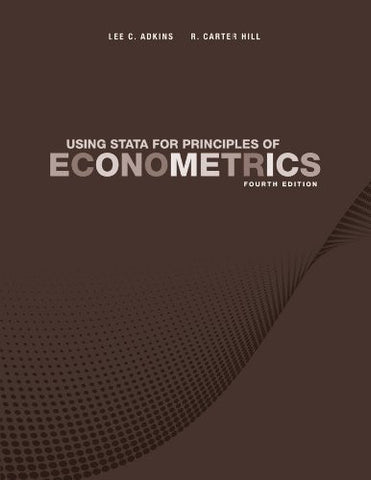# Using Stata for Principles of Econometrics

• ISBN-13: 9781118032084
• \$33.72

Only 3 left!

This is the Using Stata text for Principles of Econometrics, 4th Edition.Principles of Econometrics is an introductory book for undergraduate students in economics and finance, and can be used for MBA and first-year graduate students in many fields. The 4th Edition provides students with an understanding of why econometrics is necessary and a working knowledge of basic econometric tools.  This text emphasizes motivation, understanding and implementation by introducing very simple economic models and asking economic questions that students can answer.